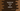# How to create an array of objects in TypeScript## TypeScript array of objects:

Array of objects can be defined in TypeScript to store data of a specific type in a sequential way. Similar to string, int or any other array, we can also have an array of objects. It is actually helpful if you are storing data in object-oriented way.

We can create an array of objects in different ways. Let me show you all one by one.

## Method 1: Inline initialization of an array of objects:

Let’s take a look at the below example:

``````let students = [{
name: 'Alex',
age: 20
},
{
name: 'Bob',
age: 21
},
{
name: 'Charlie',
age: 20
}];``````

students is an array of objects. We have not defined any type here, but it will take the type implicitely.

This is similar to:

``````let students: {name: string, age: number}[] = [{
name: 'Alex',
age: 20
},
{
name: 'Bob',
age: 21
},
{
name: 'Charlie',
age: 20
}];

console.log(students);``````

We are printing the content of students on console. It will print the below output:

``````[
{ name: 'Alex', age: 20 },
{ name: 'Bob', age: 21 },
{ name: 'Charlie', age: 20 }
]``````

## Method 2: Initializing an array of objects with optional properties:

In the above example, name and age are required for each objects. If we don’t have any of them in any object, it will throw one error.

We can mark any property optional to remove this error.

``````let students: {name: string, age?: number}[] = [{
name: 'Alex',
age: 20
},
{
name: 'Bob',
age: 21
},
{
name: 'Charlie',
}];

console.log(students);``````

In this example, the third object doesn’t have age. It will not show any error because we have marked age as optional.

## Method 3: Creating an array of objects with an interface:

In typescript, we can also create one array of objects with the type defined by an interface. The interface will hold the structure of each objects and in the array we can initialize it as this type.

``````interface Student{
name: string;
age?: number;
}

let students: Student[] = [{
name: 'Alex',
age: 20
},
{
name: 'Bob',
age: 21
},
{
name: 'Charlie',
}];

console.log(students);``````

In this example, Student interface holds one name of type string and optional age of type number. We are using the interface instead of the object type defined in previous examples.

## Method 4: Creating an array of objects with type alias:

It is almost similar to interfaces. We can use a type alias instead of an interface.

``````type Student = {
name: string;
age?: number;
}

let students: Student[] = [{
name: 'Alex',
age: 20
},
{
name: 'Bob',
age: 21
},
{
name: 'Charlie',
}];

console.log(students);``````

It will give similar result.

## Method 5: With a class:

Instead of interfaces, we can also use a class to define objects of that class type.

``````class Student{
name: string;
age?: number;

constructor(n: string, a?: number){
this.name = n;
this.age = a;
}
}

let students: Student[] = [new Student('Alex', 20), new Student('Bob', 21), new Student('Charlie')];

console.log(students);``````

Student is a class with two properties similar to the previous interface. We are creating new Student objects and inserting them to the array.

It will print:

``````[
Student { name: 'Alex', age: 20 },
Student { name: 'Bob', age: 21 },
Student { name: 'Charlie', age: undefined }
]``````

Since all are Student class objects, the print output is bit different than the previous example.

## Array operations:

We can use all array operations in an array of objects. For example, the below example uses map to iterate over the items and prints the name for each:

``````class Student{
name: string;
age?: number;

constructor(n: string, a?: number){
this.name = n;
this.age = a;
}
}

let students: Student[] = [new Student('Alex', 20), new Student('Bob', 21), new Student('Charlie')];

students.map(s => {
console.log(s.name);
})``````

It is an array, so all operations supported by typescript array can be performed.